Question

# Consider the circuit as shown. The power supply has a voltage V = 34 V and...

Consider the circuit as shown. The power supply has a voltage V = 34 V and the capacitors have capacitance C1 = C2 = C3 = C4 = 80 µF and C5 = 208 µF.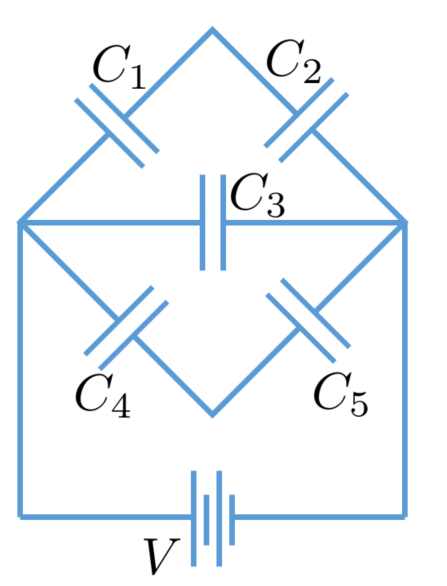(a) What is the equivalent capacitance of this circuit?

(b) What is the charge on capacitor C3?

(c) What is the voltage on capacitor C3?

(d) What is the charge on capacitor C5?

(e) What is the voltage on capacitor C5?

Use the equation for series and parallel combination for capacitors to find the equivalent capacitance and use that to find the charge and voltage on the other capacitors as shown below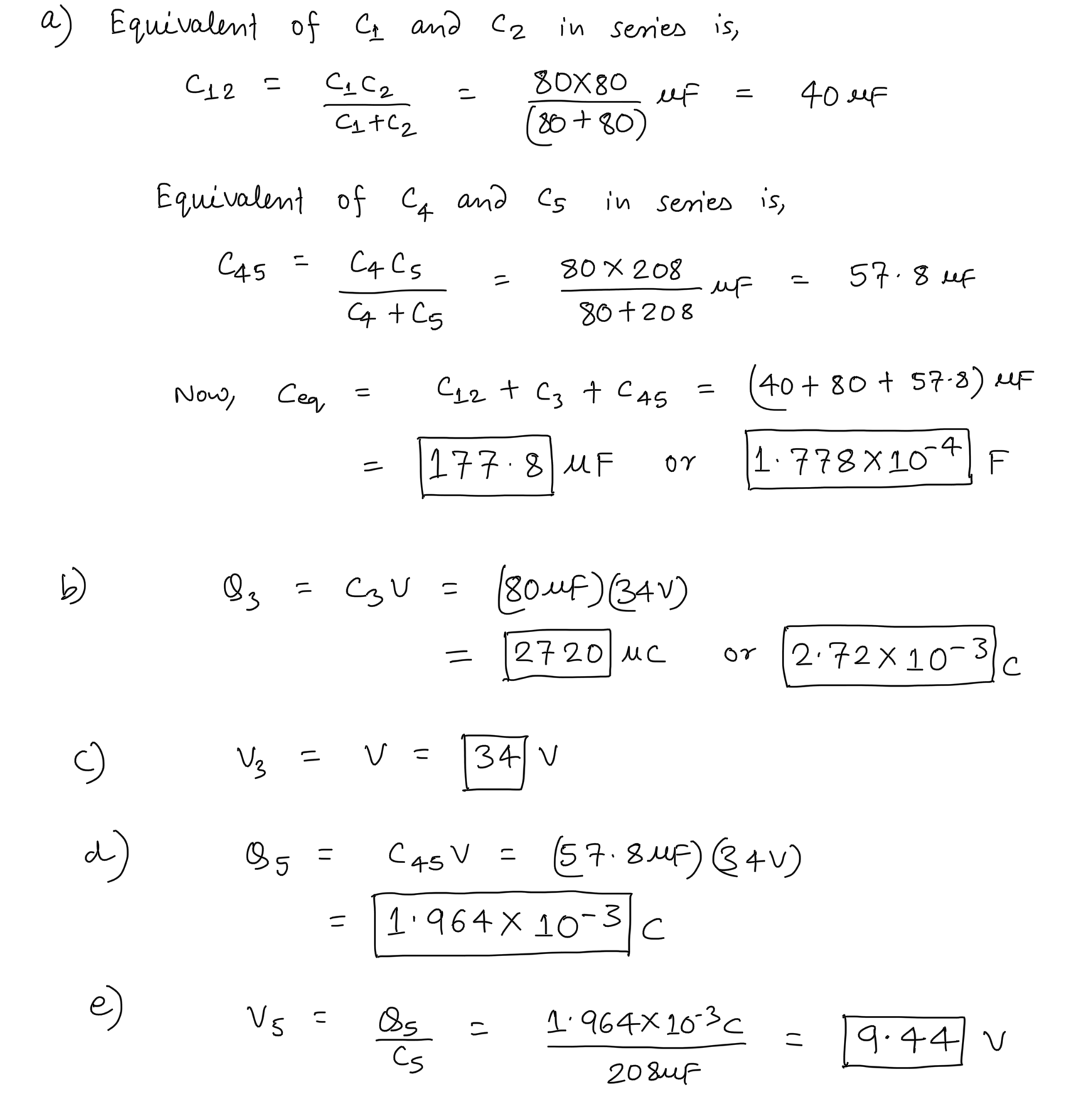#### Earn Coins

Coins can be redeemed for fabulous gifts.

Similar Homework Help Questions
• ### Four capacitors are arranged in the circuit shown in the figure. The capacitors have the values...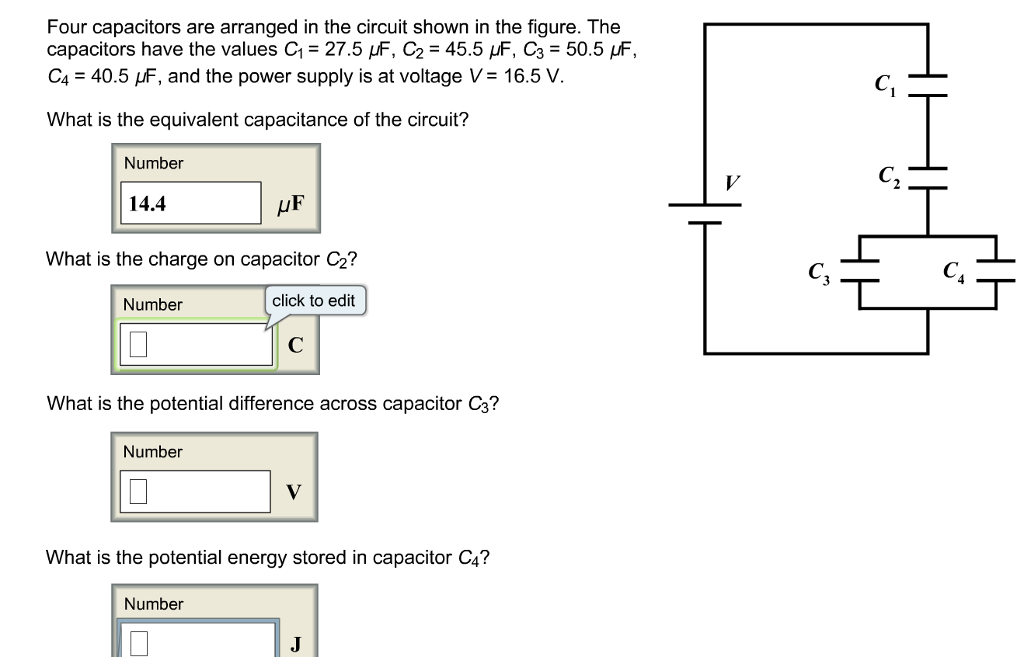Four capacitors are arranged in the circuit shown in the figure. The capacitors have the values C1 = 27.5 μF, C2 = 45.5 μF, C3 = 50.5 μF, C4 = 40.5 μF, and the power supply is at voltage V = 16.5 V. What is the equivalent capacitance of the circuit? Four capacitors are arranged in the circuit shown in the figure. The capacitors have the values C1-27.5 μF, C2 = 45.5 μF, C3 = 50.5 F, C.-40.5 and the...

• ### Vbat = C4 C5 Consider the circuit shown above. The battery provides a voltage of Vbat...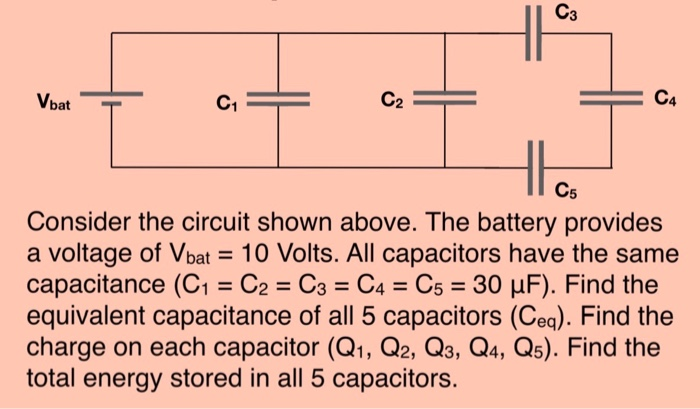Vbat = C4 C5 Consider the circuit shown above. The battery provides a voltage of Vbat = 10 Volts. All capacitors have the same capacitance (C1 = C2 = C3 = C4 = C5 = 30 uF). Find the equivalent capacitance of all 5 capacitors (Ceg). Find the charge on each capacitor (Q1, Q2, Q3, Q4, Q5). Find the total energy stored in all 5 capacitors.

• ### A circuit is constructed with five capacitors and a battery as shown. The values for the...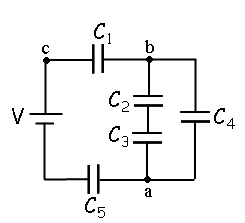A circuit is constructed with five capacitors and a battery as shown. The values for the capacitors are: C1 = C5 = 4.2 μF, C2 = 1 μF, C3 = 5.8 μF, and C4 = 4.4 μF. The battery voltage is V = 12 V. 1) What is Cab, the equivalent capacitance between points a and b?μF 2) What is Cac, the equivalent capacitance between points a and c? 3) What is Q5, the charge on capacitor C5?μC 4) What...

• ### Consider the circuit shown in which DV = 7.93 V, C1 = 5.38 µF, C2 =...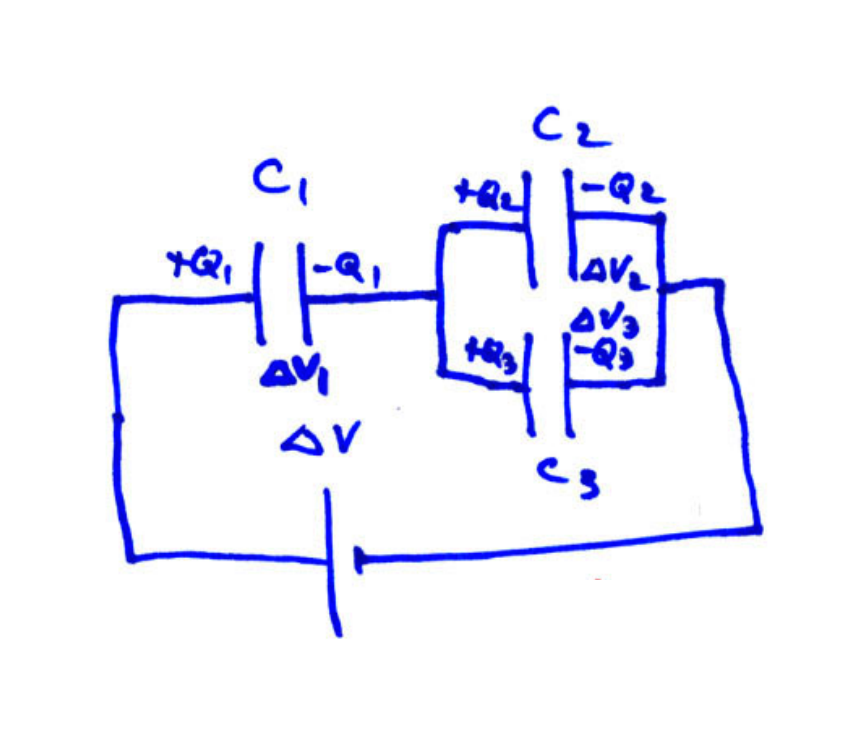Consider the circuit shown in which DV = 7.93 V, C1 = 5.38 µF, C2 = 4.24 µF and C3 = 4.89 µF. • A) Find the equivalent capacitance of the entire circuit • B) the voltage across each capacitor, and • C) the charge across each capacitor.

• ### 20 of 22 > Four capacitors are arranged in the circuit shown in the figure. The...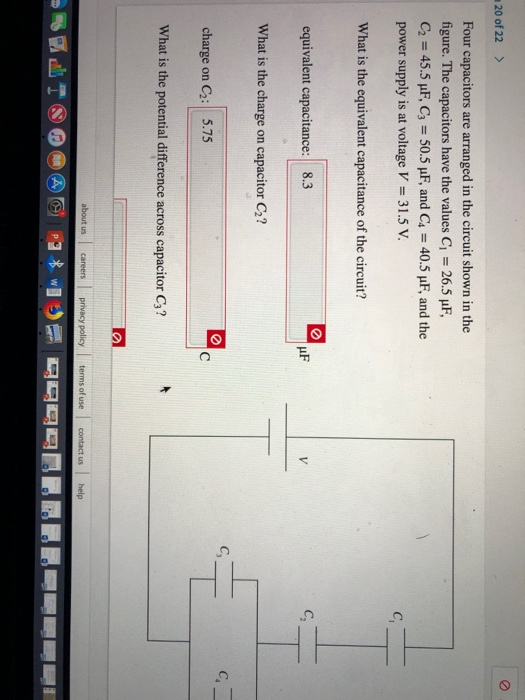20 of 22 > Four capacitors are arranged in the circuit shown in the figure. The capacitors have the values C 26.5 uF, C2 45.5 uF, C3 50.5 pF, and C4 40.5 uF, and the power supply is at voltage V 31.5 V C What is the equivalent capacitance of the circuit? C equivalent capacitance: 8.3 V What is the charge capacitor C2? on C. charge C2: 5.75 C on What is the potential difference across capacitor C3? about us...

• ### In the circuit shown in the figure the voltage of the battery is 15.0 V, and...In the circuit shown in the figure the voltage of the battery is 15.0 V, and the capacitors have the following capacitances: C1 = 1.86 mF, C2 = 3.39 mF, C3 = 3.85 mF, C4 = 5.79 mF. What is the equivalent capacitance of the four capacitors?

• ### A circuit is constructed with five capacitors and a battery as shown. The values for...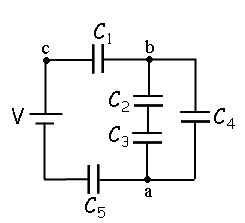A circuit is constructed with five capacitors and a battery as shown. The values for the capacitors are: C1 = C5 = 3.9 μF, C2 = 1.9 μF, C3 = 7.6 μF, and C4 = 2.2 μF. The battery voltage is V = 12 V. 4)What is Q2, the charge on C2? 5)What is Q1, the charge on capacitor C1? C1 b HH Cz C4 Ca HH a C5

• ### Problem 5: (24 points) Consider the circuit shown below. Assume C1 = 30 nF, C2 =...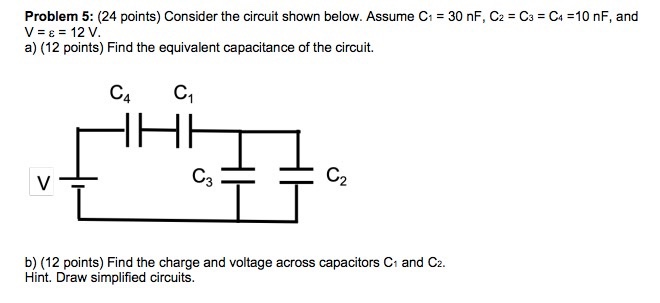Problem 5: (24 points) Consider the circuit shown below. Assume C1 = 30 nF, C2 = C3 = C4 = 10 nF, and V = e = 12 V. a) (12 points) Find the equivalent capacitance of the circuit. c, c, AHA v1 C3 = b) (12 points) Find the charge and voltage across capacitors C1 and C2. Hint. Draw simplified circuits.

• ### The figure shows a circuit with an ideal battery and set of capacitors. The battery has...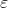The figure shows a circuit with an ideal battery and set of capacitors. The battery has an emf =12 V. The capacitors have capacitances C1 = C2 = C3 = C4 = 6 nF. (a) What is the equivalent capacitance of the entire network of capacitors? (b) What is the electrical energy stored in the capacitor with capacitance C2? We were unable to transcribe this imageProblem 6 C. The figure shows a circuit with an ideal battery and set of...